# Algorithm Design Manual Chapter 5

## Book Notes

### 5.1 Flavors of Graphs

• Undirected vs. Directed
• Weighted vs. Unweighted
• Simple vs. Non-simple
• Sparse vs. Dense
• Cyclic vs. Acyclic
• Embedded vs. Topological
• Implicit vs. Explicit
• Labeled vs. Unlabeled

### 5.2 Data Structures for Graphs

• Adjacency Matrix: We can represent G using an n×n matrix M, where element M[i, j] = 1 if(i, j) is an edge of G, and 0 if it isn’t.
• Adjacency Lists: We can more efficiently represent sparse graphs by using linked lists to store the neighbors adjacent to each vertex.

Adjacency lists are the right data structure for most applications of graphs.

#define MAXV 1000  // maximum number of vertices

typedef struct {
int weight;  // edge weight, if any
struct edgenode *next;  // next edge in list
} edgenode;

typedef struct {
edgenode *edges[MAXV + 1];   // adjacency info
int degree[MAXV + 1];  // outdegree of each vertex
int nvertices;  // number of vertices in graph
int nedges;  // number of edges in graph
bool directed;  // is the graph directed
} graph;

void initialize_graph(graph *g, bool directed) {
int i;
g->nvertices = 0;
g->nedges = 0;
g->directed = directed;
for (i = 1; i <= NMAX; ++i) {
g->degree[i] = 0;
g->edges[i] = NULL;
}
}

void insert_edge(graph *g, int x, int y, bool directed) {
edgenode *p;
p = new edgenode;
p-> weight = 0;
p->y = y;
p->next = g->edges[x];
g->edges[x] = p;
g->degree[x]++;
if (directed == false) {
insert_edge(g, y, x, true);
} else {
g->nedges++;
}
}

void read_graph(graph *g, bool directed) {
int i;
int m;
int x, y;
initialize_graph(g, directed);
scanf("%d %d", &(g->nvertices), &m);
for (i = 1; i <= m; ++i) {
scanf("\$d %d", &x, &y);
insert_edge(g, x, y, directed);
}
}

print_graph(graph *g) {
int i;
edgenode *p;
for (i = 1; i <= g->nvertices; ++i) {
printf("%d: ", i);
p = g->edges[i];
while (p != NULL) {
printf("%d ", p->y);
p = p->next;
}
printf("\n");
}
}


### 5.5 Traversing a Graph

The key idea behind graph traversal is to mark each vertex when we first visit it and keep track of what we have not yet completely explored. Although bread crumbs or unraveled threads have been used to mark visited places in fairy-tale mazes, we will rely on Boolean flags or enumerated types.

Each vertex will exist in one of three states:

• undiscovered– the vertex is in its initial, virgin state.
• discovered– the vertex has been found, but we have not yet checked out all its incident edges.
• processed– the vertex after we have visited all its incident edges.

    bool processed[MAXV+1]; /* which vertices have been processed */
bool discovered[MAXV+1]; /* which vertices have been found */
int parent[MAXV+1]; /* discovery relation */

initialize_search(graph *g)
{
int i; /* counter */
for (i=1; i<=g->nvertices; i++) {
processed[i] = discovered[i] = FALSE;
parent[i] = -1;
}
}

bfs(graph *g, int start)
{
queue q; /* queue of vertices to visit */
int v; /* current vertex */
int y; /* successor vertex */
edgenode *p; /* temporary pointer */
init_queue(&q);
enqueue(&q,start);
discovered[start] = TRUE;
while (empty_queue(&q) == FALSE) {
v = dequeue(&q);
process_vertex_early(v);
processed[v] = TRUE;
p = g->edges[v];
while (p != NULL) {
y = p->y;
if ((processed[y] == FALSE) || g->directed)
process_edge(v,y);
if (discovered[y] == FALSE) {
enqueue(&q,y);
discovered[y] = TRUE;
parent[y] = v;
}
p = p->next;
}
process_vertex_late(v);
}
}

find_path(int start, int end, int parents[])
{
if ((start == end) || (end == -1))
printf("\n%d",start);
else {
find_path(start,parents[end],parents);
printf(" %d",end);
}
}


Because vertices are discovered in order of increasing distance from the root, this tree has a very important property. The unique tree path from the root to each node x∈V uses the smallest number of edges (or equivalently, intermediate nodes) possible on any root-to-xpath in the graph.

There are two points to remember when using breadth-first search to find a shortest path fromxtoy: First, the shortest path tree is only useful if BFS was performed with x as the root of the search. Second, BFS gives the shortest path only if the graph is unweighted.

### 5.7 Applications of Breadth-First Search

Properly implemented using adjacency lists, any such algorithm is destined to be linear, since BFS runs in O(n+m) time on both directed and undirected graphs. This is optimal, since it is as fast as one can hope to read any n-vertex, m-edge graph.

### 5.8 Depth-First Search

The difference between BFS and DFS results is in the order in which they explore vertices. This order depends completely upon the container data structure used to store the discovered but not processed vertices.

• Queue– By storing the vertices in a first-in, first-out (FIFO) queue, we explore the oldest unexplored vertices first. Thus our explorations radiate out slowly from the starting vertex, defining a breadth-first search.
• Stack– By storing the vertices in a last-in, first-out (LIFO) stack, we explore the vertices by lurching along a path, visiting a new neighbor if one is available, and backing up only when we are surrounded by previously discovered vertices. Thus, our explorations quickly wanderaway from our starting point, defining a depth-first search.

DFS organizes vertices by entry/exit times, and edges into tree and back edges. This organization is what gives DFS its real power.

Implementation

The beauty of implementingdfsrecursively is that recursion eliminates the need to keep an explicit stack:

dfs(graph *g, int v)
{
edgenode *p; /* temporary pointer */
int y; /* successor vertex */
if (finished) return; /* allow for search termination */
discovered[v] = TRUE;
time = time + 1;
entry_time[v] = time;
process_vertex_early(v);
p = g->edges[v];
while (p != NULL) {
y = p->y;
if (discovered[y] == FALSE) {
parent[y] = v;
process_edge(v,y);
dfs(g,y);
}
else if ((!processed[y]) || (g->directed))
process_edge(v,y);
if (finished) return;
p = p->next;
}
process_vertex_late(v);
time = time + 1;
exit_time[v] = time;
processed[v] = TRUE;
}


### 5.9 Applications of Depth-First Search

#### Finding Cycles

But any back edge going from x to an ancestorycreates a cycle with the tree path fromytox. Such a cycle is easy to find using dfs:

process_edge(int x, int y)
{
if (parent[x] != y) { /* found back edge! */
printf("Cycle from %d to %d:",y,x);
find_path(y,x,parent);
printf("\n\n");
finished = TRUE;
}
}


#### Articulation Vertices

Observe that there is a single point of failure—a single vertex whose deletion disconnects a connected component of the graph. Such a vertex is called an articulation vertex or cut-node.

More robust graphs without such a vertex are said to be biconnected.

Temporarily delete each vertex v, and then do a BFS or DFS traversal of the remaining graph to establish whether it is still connected. The total time fornsuch traversals is O(n(m+n)). There is a clever linear-time algorithm, however, that tests all the vertices of a connected graph using a single depth-first search.

Let reachable_ancestor[v] denote the earliest reachable ancestor of vertex v, meaning the oldest ancestor ofvthat we can reach by a combination of tree edges and back edges. Initially, reachable_ancestor[v] = v:

int reachable_ancestor[MAXV+1]; /*earliestreachableancestorofv*/
int tree_out_degree[MAXV+1];  /* DFStree outdegree ofv*/
process_vertex_early(int v)
{
reachable_ancestor[v] = v;
}


We update reachable_ancestor[v] whenever we encounter a back edge that takes us to an earlier ancestor than we have previously seen. The relative age/rank of our ancestors can be determined from their entry_time’s:

process_edge(int x, int y)
{
int class; /* edge class */
class = edge_classification(x,y);
if (class == TREE)
tree_out_degree[x] = tree_out_degree[x] + 1;
if ((class == BACK) && (parent[x] != y)) {
if (entry_time[y] < entry_time[ reachable_ancestor[x] ] )
reachable_ancestor[x] = y;
}
}


The key issue is determining how the reachability relation impacts whether vertexv is an articulation vertex. There are three cases:

• Root cut-nodes– If the root of the DFS tree has two or more children, it must be an articulation vertex. No edges from the subtree of the second child can possibly connect to the subtree of the first child.
• Bridge cut-nodes– If the earliest reachable vertex fromvis v, then deleting the single edge (parent[v],v) disconnects the graph. Clearlyparent[v] must be an articulation vertex, since it cuts v from the graph. Vertex vis also an articulation vertex unless it is a leaf of the DFS tree. For any leaf, nothing falls off when you cut it.
• Parent cut-nodes– If the earliest reachable vertex fromvis the parent of v, then deleting the parent must severvfrom the tree unless the parent is the root.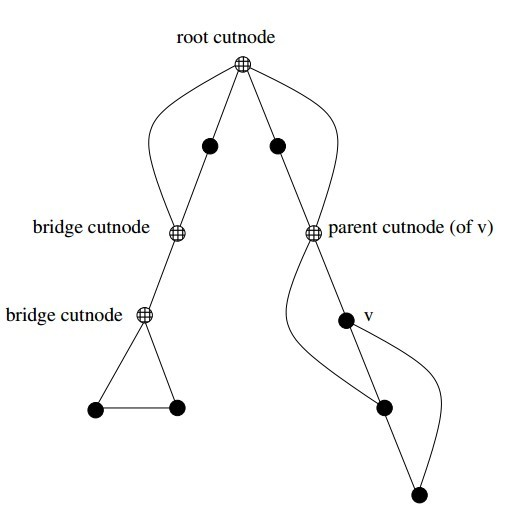The routine below systematically evaluates each of the three conditions as we back up from the vertex after traversing all outgoing edges. We use entry_time[v] to represent the age of vertex v. The reachability time time_v calculated below denotes the oldest vertex that can be reached using back edges.

process_vertex_late(int v)
{
bool root; /* is the vertex the root of the DFS tree? */
int time_v; /* earliest reachable time for v */
int time_parent; /* earliest reachable time for parent[v] */
if (parent[v] < 1) { /* test if v is the root */
if (tree_out_degree[v] > 1)
printf("root articulation vertex: %d \n",v);
return;
}
root = (parent[parent[v]] < 1); /* is parent[v] the root? */
if ((reachable_ancestor[v] == parent[v]) && (!root))
printf("parent articulation vertex: %d \n",parent[v]);
if (reachable_ancestor[v] == v) {
printf("bridge articulation vertex: %d \n",parent[v]);
if (tree_out_degree[v] > 0) /* test if v is not a leaf */
printf("bridge articulation vertex: %d \n",v);
}
time_v = entry_time[reachable_ancestor[v]];
time_parent = entry_time[ reachable_ancestor[parent[v]] ];
if (time_v < time_parent)
reachable_ancestor[parent[v]] = reachable_ancestor[v];
}


We can alternately talk about reliability in terms of edge failures instead of vertex failures.

In fact all bridges can be identified in the same O(n+m) time. Edge (x, y) is a bridge if (1) it is a tree edge, and (2) no back edge connects from yor below toxor above. This can be computed with a minor modification of the reachable_ancestor function.

### 5.10 Depth-First Search on Directed Graphs

For directed graphs, depth-first search labelings can take on a wider range of possibilities. Indeed, all four of the edge cases in Figure below can occur in traversing directed graphs.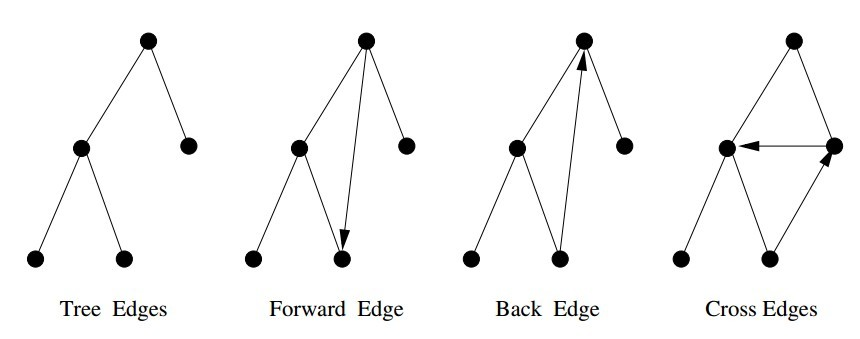The correct labeling of each edge can be readily determined from the state, discovery time, and parent of each vertex, as encoded in the following function:

int edge_classification(int x, int y)
{
if (parent[y] == x) return(TREE);
if (discovered[y] && !processed[y]) return(BACK);
if (processed[y] && (entry_time[y]>entry_time[x])) return(FORWARD);
if (processed[y] && (entry_time[y]<entry_time[x])) return(CROSS);
printf("Warning: unclassified edge (%d,%d)\n",x,y);
}


#### Strongly Connected Components

A directed graph isstrongly connectedif there is a directed path between any two vertices.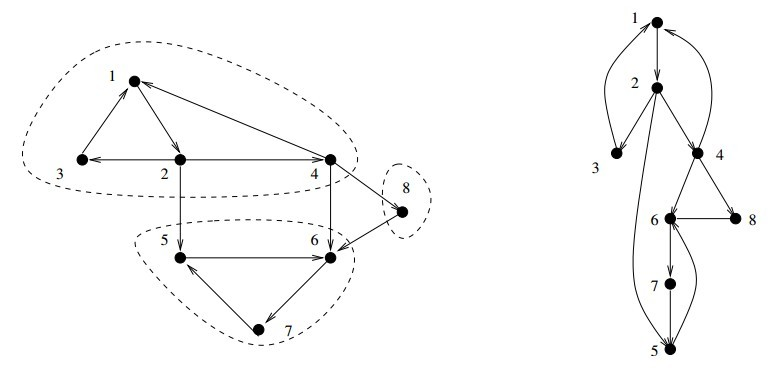The algorithm is based on the observation that it is easy to find a directed cycle using a depth-first search, since any back edge plus the down path in the DFS tree gives such a cycle. All vertices in this cycle must be in the same strongly connected component. Thus, we can shrink (contract) the vertices on this cycle down to a single vertex representing the component, and then repeat. This process terminates when no directed cycle remains, and each vertex represents a different strongly connected component.

We update our notion of the oldest reachable vertex in response to (1) nontree edges and (2) backing up from a vertex.

strong_components(graph *g)
{
int i; /* counter */
for (i=1; i<=(g->nvertices); i++) {
low[i] = i;
scc[i] = -1;
}
components_found = 0;
init_stack(&active);
initialize_search(&g);
for (i=1; i<=(g->nvertices); i++)
if (discovered[i] == FALSE) {
dfs(g,i);
}
}


Define low[v]to be the oldest vertex known to be in the same strongly connected component asv. This vertex is not necessarily an ancestor, but may also be a distant cousin of v because of cross edges. Cross edges that point vertices from previous strongly connected components of the graph cannot help us, because there can be no way back from them tov, but otherwise cross edges are fair game. Forward edges have no impact on reachability over the depth-first tree edges, and hence can be disregarded:

int low[MAXV+1]; /* oldest vertex surely in component of v */
int scc[MAXV+1]; /* strong component number for each vertex */
process_edge(int x, int y)
{
int class; /* edge class */
class = edge_classification(x,y);
if (class == BACK) {
if (entry_time[y] < entry_time[ low[x] ] )
low[x] = y;
}
if (class == CROSS) {
if (scc[y] == -1) /* component not yet assigned */
if (entry_time[y] < entry_time[ low[x] ] )
low[x] = y;
}
}


A new strongly connected component is found whenever the lowest reachable vertex fromvis v. If so, we can clear the stack of this component. Otherwise, we give our parent the benefit of the oldest ancestor we can reach and backtrack:

  process_vertex_early(int v)
{
push(&active,v);
}

process_vertex_late(int v)
{
if (low[v] == v) { /* edge (parent[v],v) cuts off scc */
pop_component(v);
}
if (entry_time[low[v]] < entry_time[low[parent[v]]])
low[parent[v]] = low[v];
}

pop_component(int v)
{
int t; /* vertex placeholder */
components_found = components_found + 1;
scc[ v ] = components_found;
while ((t = pop(&active)) != v) {
scc[ t ] = components_found;
}
}


## Exercises

### 5

Give a linear algorithm to compute the chromatic number of graphs where each vertex has degree at most 2. Must such graphs be bipartite?

### 7

Given pre-order and in-order traversals of a binary tree, is it possible to reconstruct the tree? If so, sketch an algorithm to do it. If not, give a counterexample. Repeat the problem if you are given the pre-order and post-order traversals.

preorder = {1,1}
inorder = {1,1}


  1                     1
/           or          \
1                         1


struct Node {
int val;
struct Node* left;
struct Node* right;
Node(int val_in) {
val = val_in;
left = NULL;
right = NULL;
}
};

const int kMax = 256;
int map_index[kMax];

void MapToIndex(int inorder[], int n) {
for (int i = 0; i < n; ++i) {
map_index[inorder[i]] = i;
}
}

Node *BuildInorderPreorder(in in[], in pre[], int n, int offset) {
if (n == 0) {
return NULL:
}
int root_val = pre;
int i = map_index[root_val] - offset;
Node *root = new Node(root_val);
root->left = BuildInorderPreorder(in, pre+1, i, offset);
root->right = BuildInorderPreorder(in+i+1, pre+i+1, offset+i+1);
return root;
}

• 给予 pre-order and post-order traversals, 不能重构 binary search tree.

### 12

The square of a directed graph G = (V,E) is the graph G2 = (V,E2) such that (u,w)∈E2 iff there exists v∈V, such that (u,v)∈E and (u,w)∈E; i.e., there is a path of exactly two edges from u to w. square of a graph Give efficient algorithms for both adjacency lists and matrices.

MakeSquareGraph(G, n)
for i=1 to n
for j=1 to n
G2[i][j] = 0
for i=1 to n
for j=1 to n
if (G[i][j] == 1)
for k=1 to n
if (G[j][k] == 1)
G2[i][k] = 1
return G2


### 18

Consider a set of movies $$M_1, M_2, \ldots, M_k$$. There is a set of customers, each one of which indicates the two movies they would like to see this weekend. Movies are shown on Saturday evening and Sunday evening. Multiple movies may be screened at the same time. You must decide which movies should be televised on Saturday and which on Sunday, so that every customer gets to see the two movies they desire. Is there a schedule where each movie is shown at most once? Design an efficient algorithm to find such a schedule if one exists.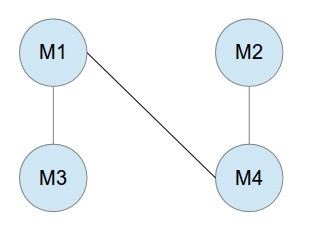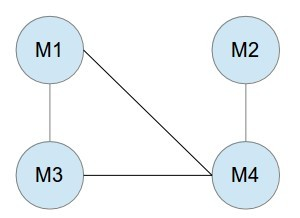### 23

Your job is to arrange n ill-behaved children in a straight line, facing front. You are given a list of m statements of the form i hates j. If i hates j, then you do not want put i somewhere behind j, because then i is capable of throwing something at j.

1. Give an algorithm that orders the line, (or says that it is not possible) in O(m + n) time.
2. Suppose instead you want to arrange the children in rows such that if i hates j, then i must be in a lower numbered row than j. Give an efficient algorithm to find the minimum number of rows needed, if it is possible.
3. 创建一幅有向图,顶点代表孩子,有向边 E(i,j)代表孩子 i hates 孩子 j;
4. topological sort 得到队列，或 BFS 时发现环，证明不可能。只 BFS 遍历一次， O(m + n)。
5. 如题 1 创建有向图;
6. 作 DFS 遍历，用遍历的 level 作为行号。

### 31

Which data structures are used in depth-first and breath-first search?

1. BFS:使用 queue
2. DFS:使用 stack,通常使用递归代替 stack.

### 32

Write a function to traverse binary search tree and return the ith node in sorted order.

struct Node {
int val;
struct Node* left;
struct Node* right;
Node(int val_in) {
val = val_in;
left = NULL;
right = NULL;
}
};

bool FindIthElementCore(struct Node *root, int ith, int *index, int *value) {
if (root == NULL) {
return false;
}
if (FindIthElementCore(root->left, ith, index, value)) {
return true;
}
cout << ith << ": " << *index << ": " << root->val << endl;
if (ith == *index) {
*value = root->val;
return true;
}
(*index)++;
if (FindIthElementCore(root->right, ith, index, value)) {
return true;
} else {
return false;
}
}

bool FindIthElement(struct Node *root, int ith, int *value) {
int start = 0;
return FindIthElementCore(root, ith, &start, value);
}